POSITION 14.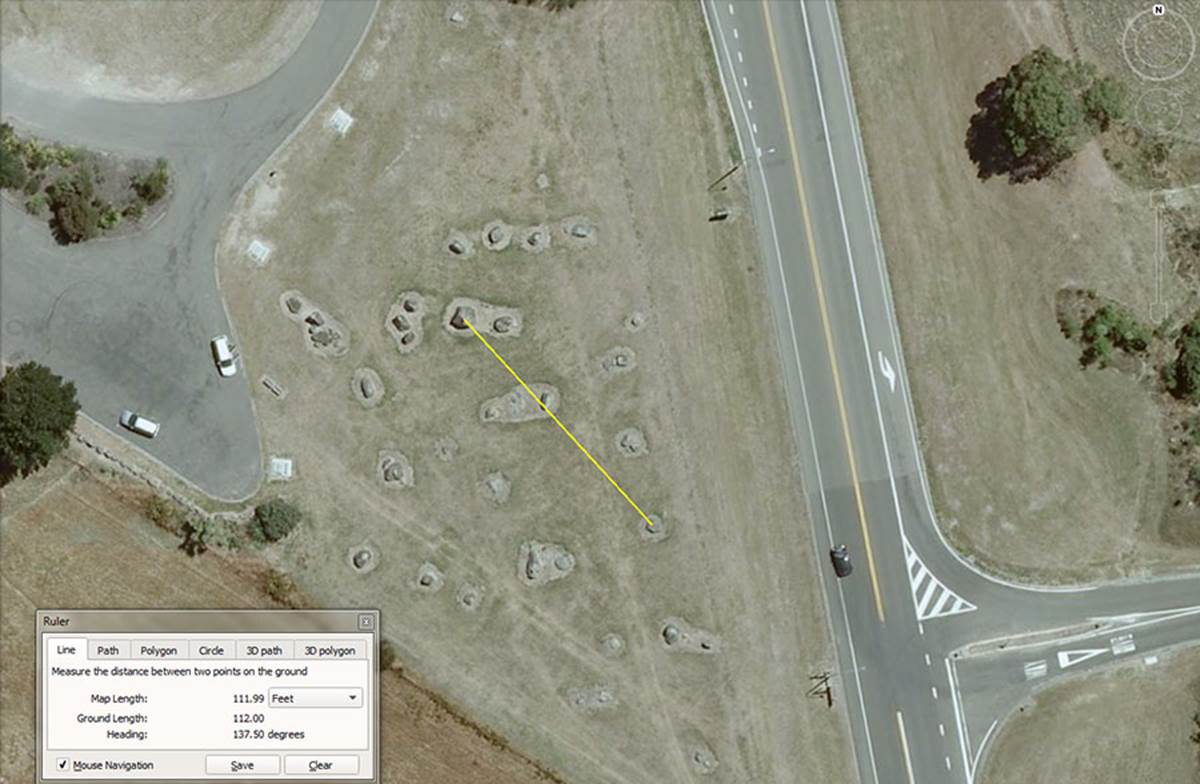This position sits 112-feet from the hubstone on an angle of 137.5-degrees. For tutorial purposes by a learned master, the length codes could also be read as 112.5 & 113.4-feet respectively, possibly even 115.2-feet.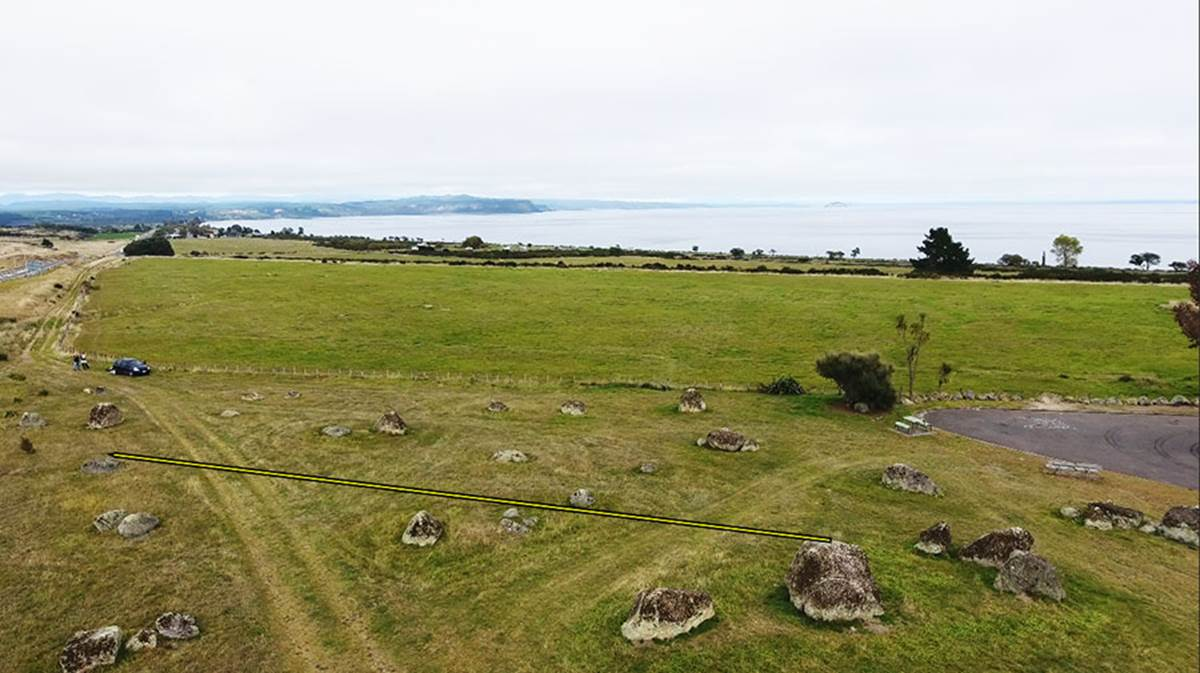POSITION 15.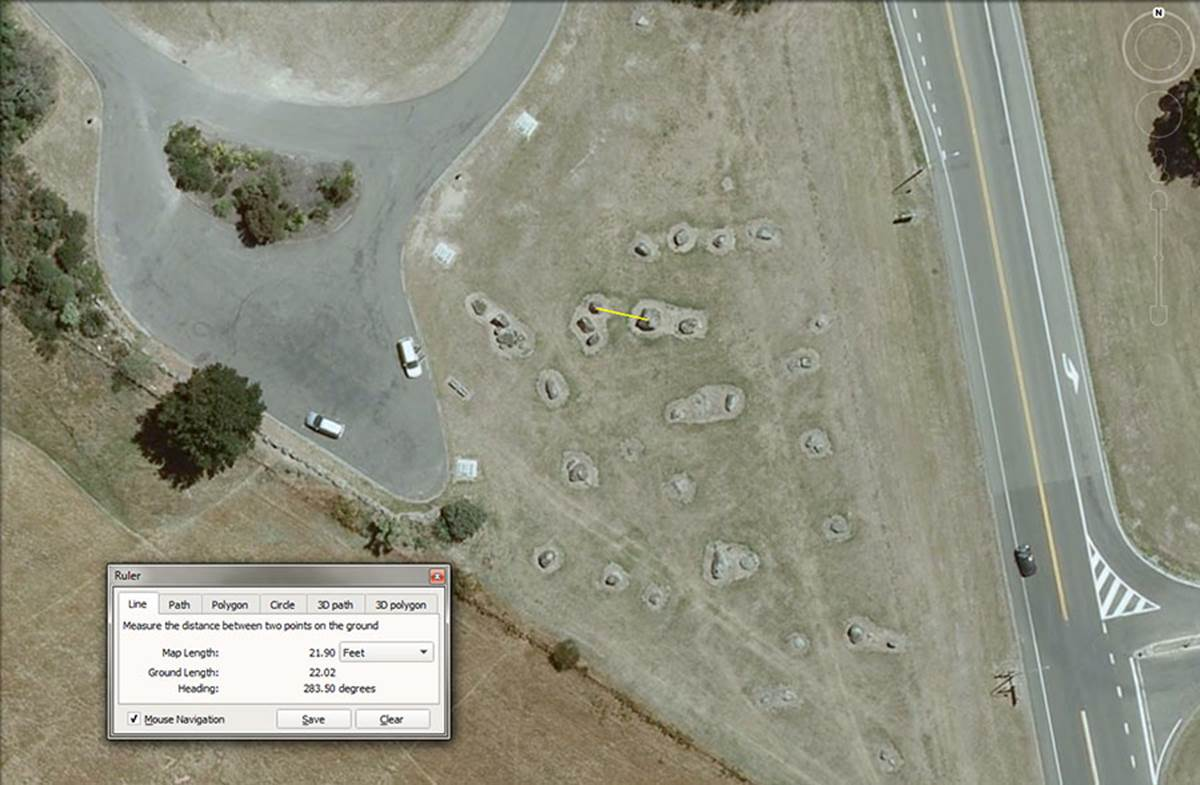This position sits 22-feet from the centre hubstone at an angle of 283.5-degrees. Tutorials for the lengths 21, 21.6, 22.5, 22.68, 23.04, 24, 24.3, 24.75 and 24.8832 feet respectively were also within the scope of this position to provide.

The second obelisk of this positon sits 25.92-feet (Precession of the Equinoxes coding) from the hubstone on an angle of 268.8-degrees (navigation, lunar). The angle of 270-degrees is also achievable as is the length 26.25-feet.

The third obelisk in this cluster sits 24-feet from the hubstone on an angle of 250-degrees. A coded angle of 252-degrees is also achievable, as are lengths of 24.3, 24.750 & 24.883.2-degrees respectively.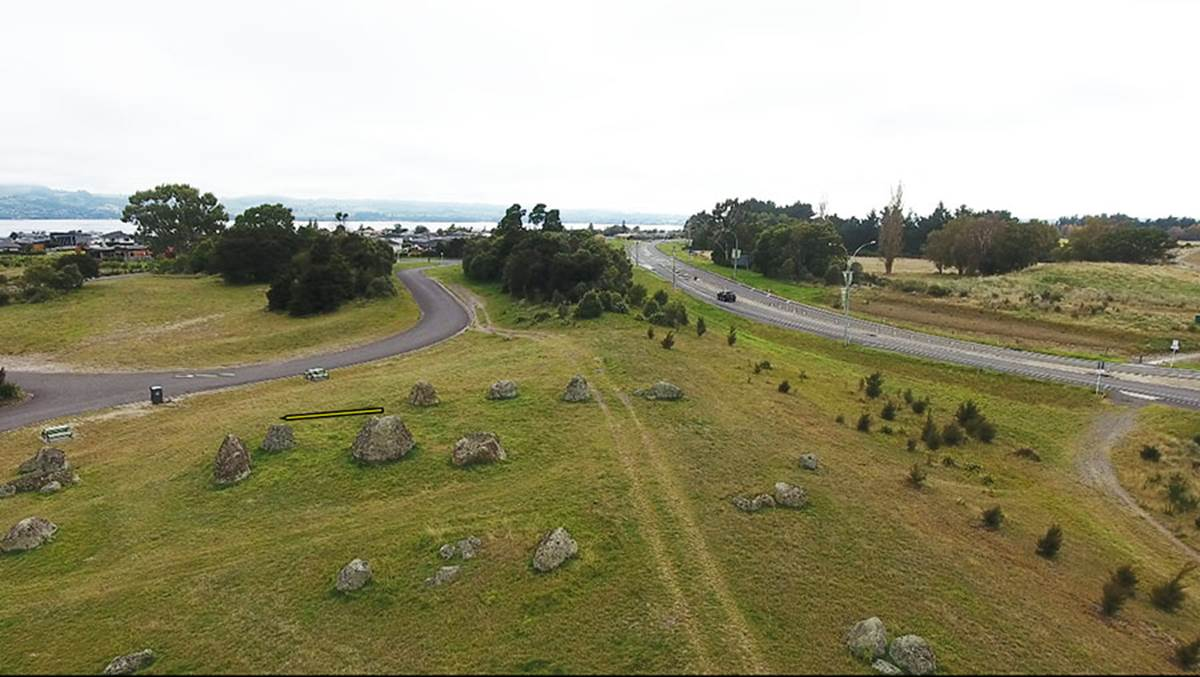POSITION 16.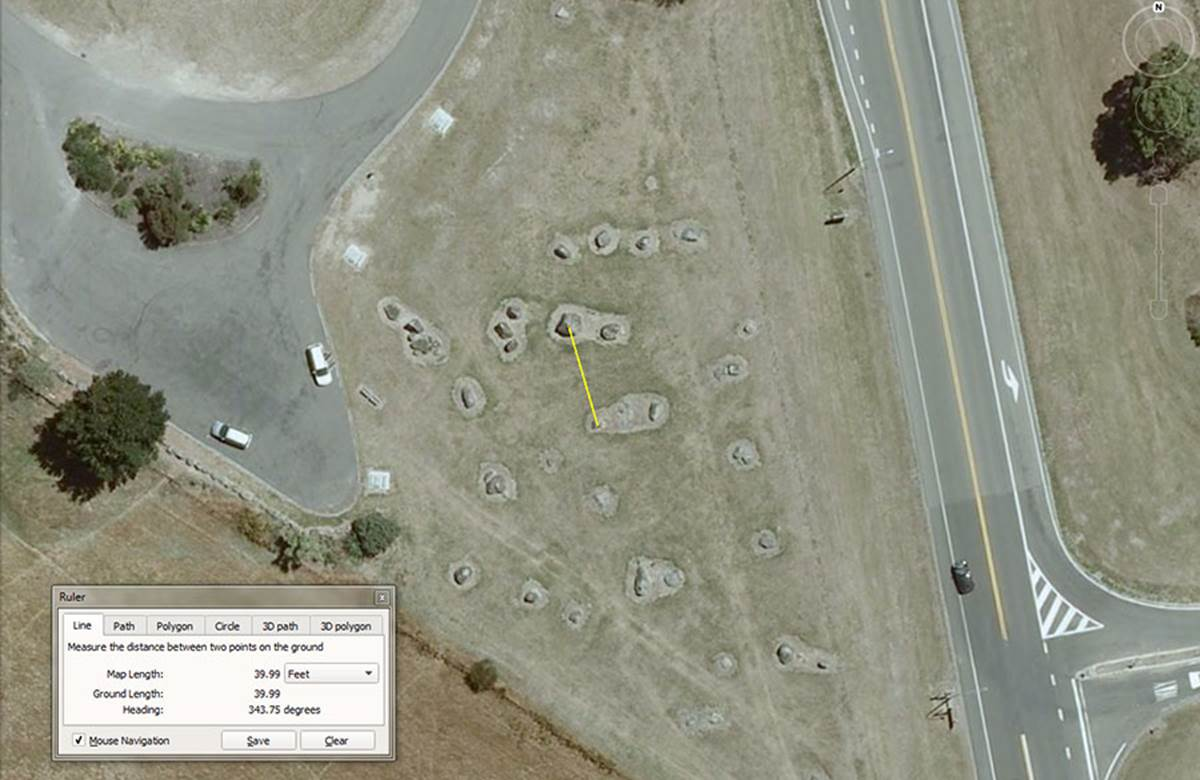This position is 40-feet from the hubstone with a coded return angle of 343.75-degrees (equatorial size of the Earth coding). The sum of 34.375-miles would be ½ of a degree of arc under the 5280-miles system of equatorial circumference coding, with the Earth considered to be 24750-miles around.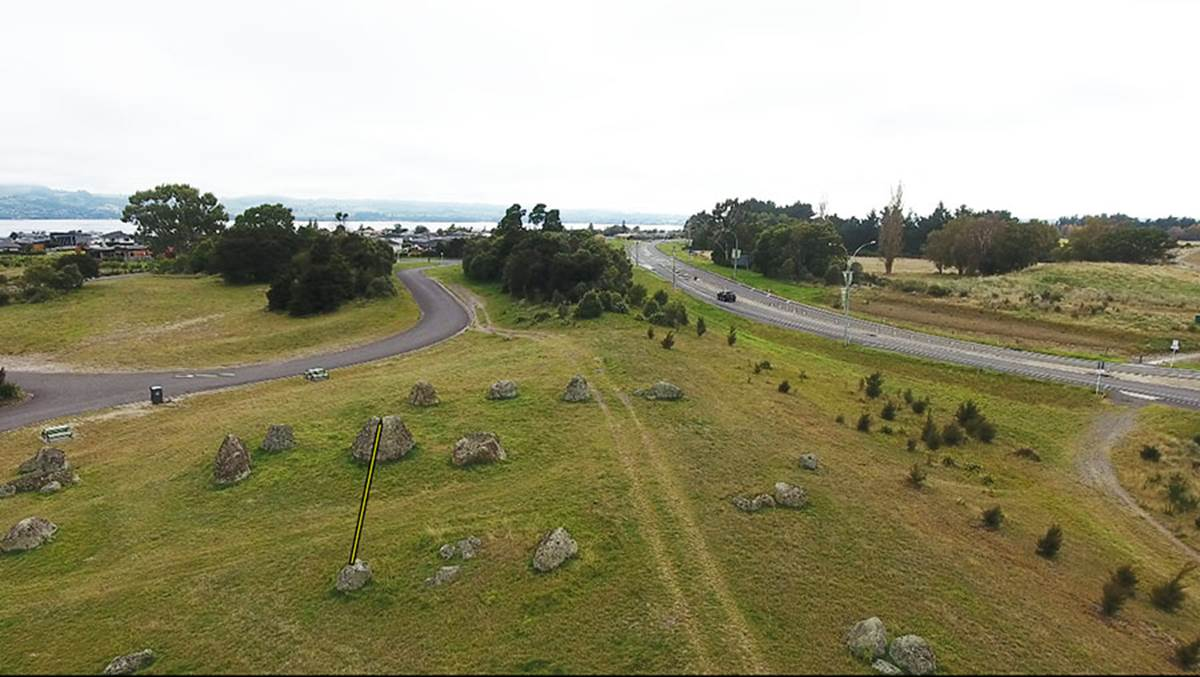POSITION 17.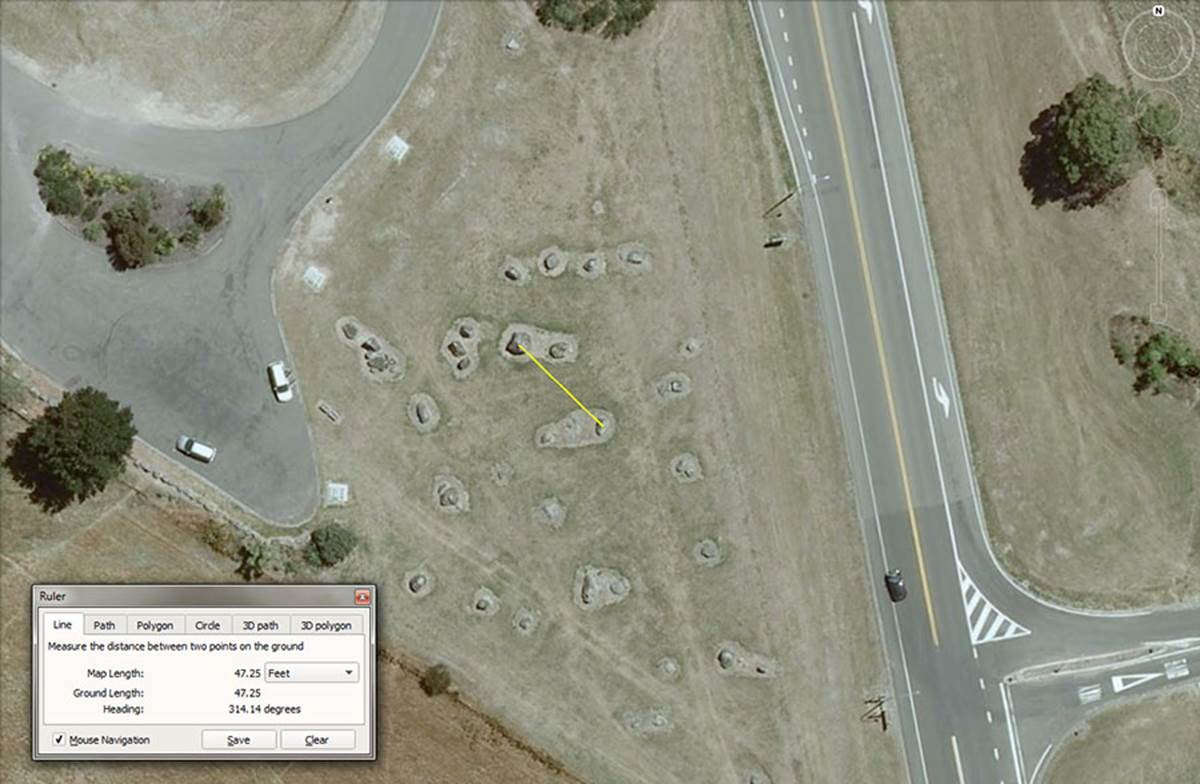The distance to this boulder is 47.25-feet, with a return angle back to the hubstone of 314.16-degrees. The position would have been very effective for teaching initiates the several forms of the PI ratio in use in ancient times for various kinds if calculations. There was use of 1728/550ths (3.141818182); 22/7th (3.142857143); 3.15 and 3.125, depending upon the type of calculation being computed.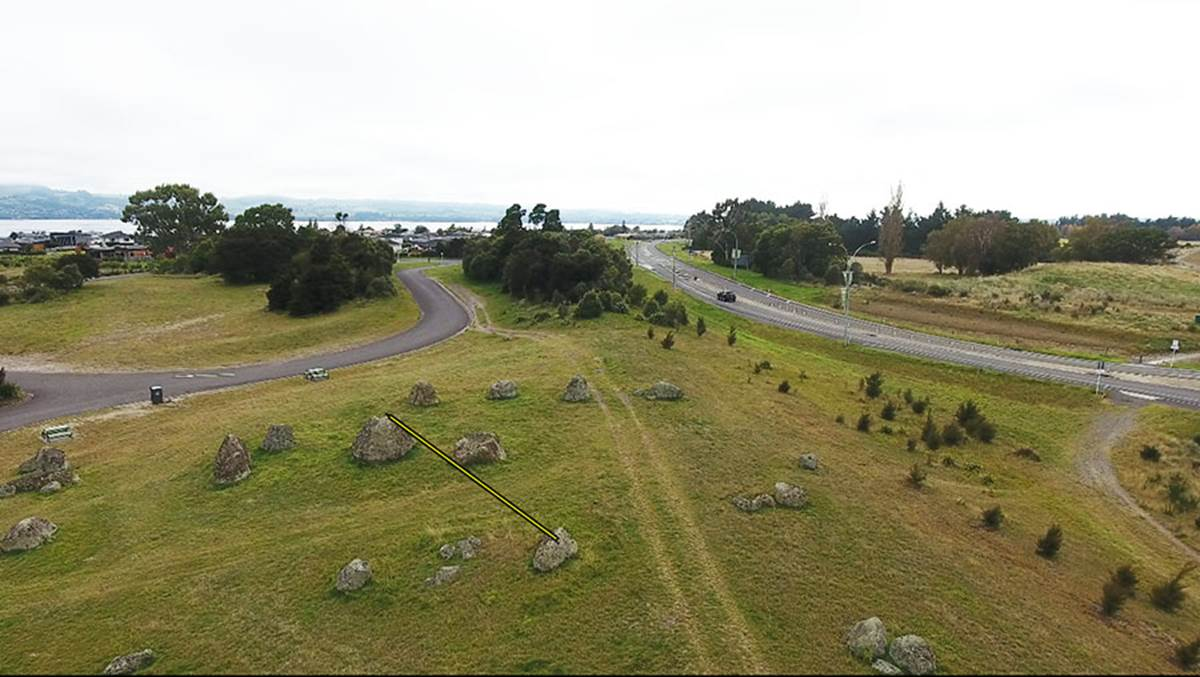The boulder cairn, situated between the two obelisks of positions 16 & 17, sits 40.5-feet (lunar coding & Precession of the Equinoxes, etc.) from the hubstone at a coded angle of 145.8-degrees (navigation by, what became a Roman method).

POSITION 18.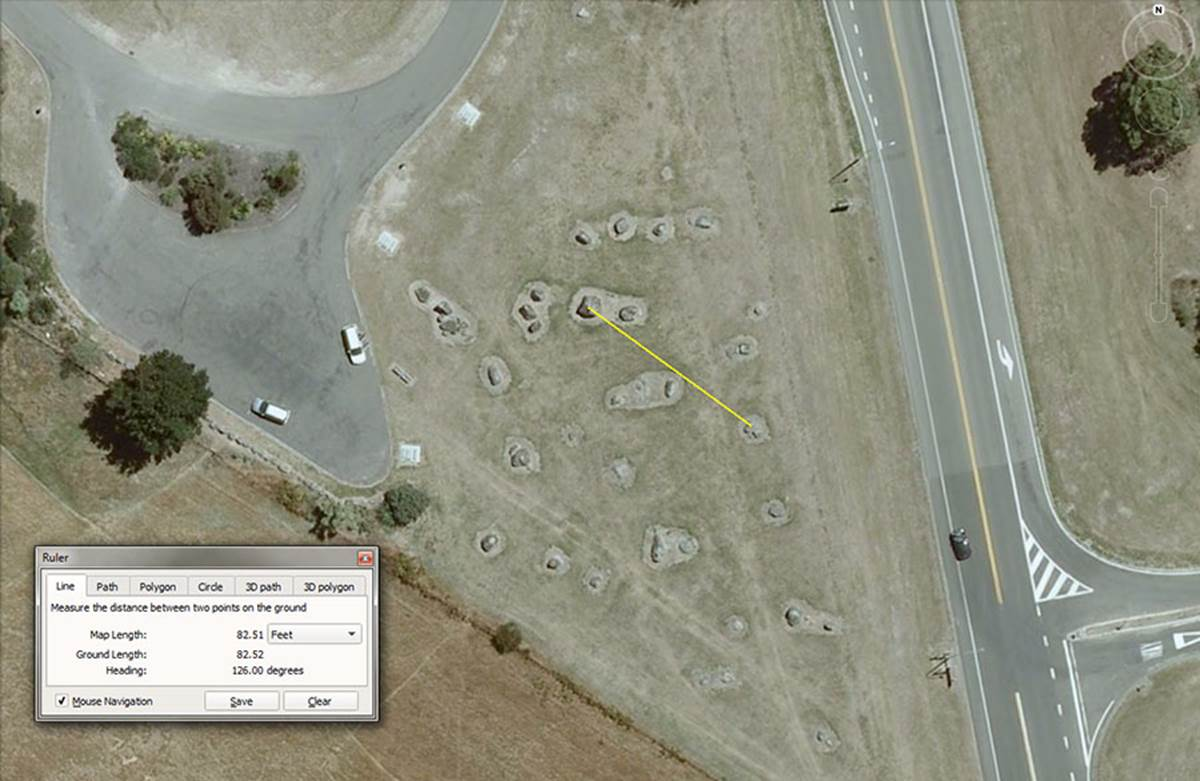This double set of stones sits 82.5-feet (navigation using the 5280-feet mile) out from the hubstone at a coded angle of 126-degrees (the Greek foot, Assyrian cubit, Hebrew Reed, Greek mile, etc.).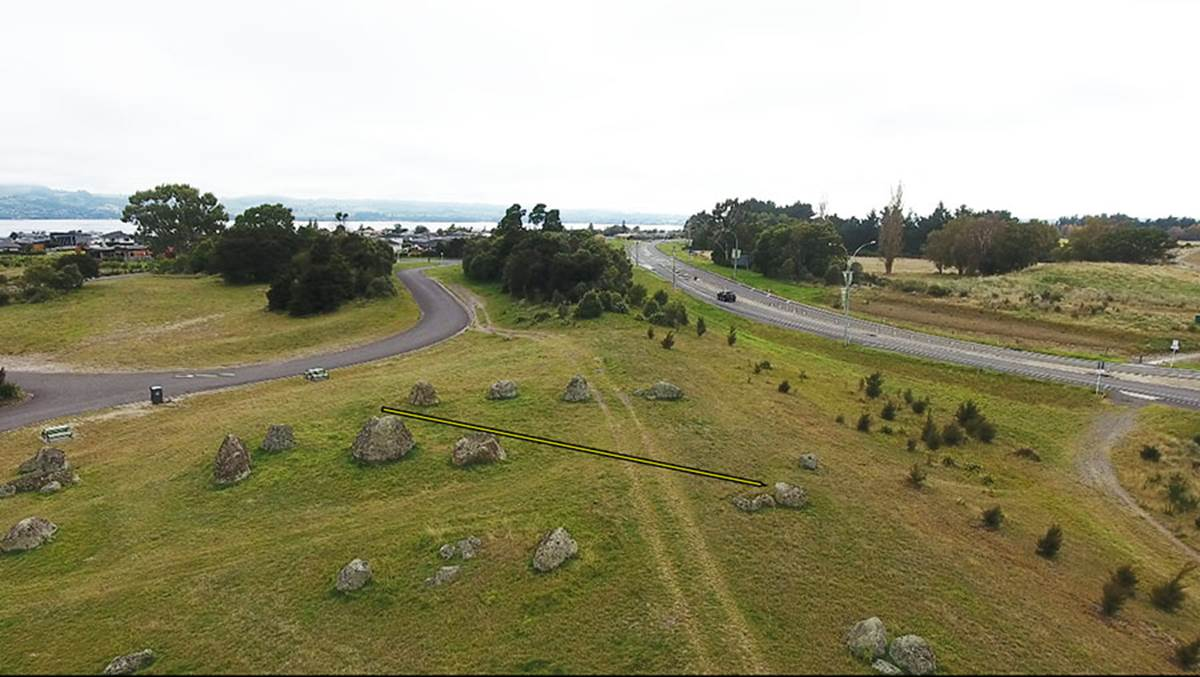The second of the two boulders could carry the distance code of 86.4-feet.

Whether coincidental or not, the sun has a diameter of 864,000-miles.

Continue